# KR101394151B1 - Apparatus and method for encoding image using a psycho-visual characteristic - Google Patents

Apparatus and method for encoding image using a psycho-visual characteristic Download PDF

## Info

Publication number
KR101394151B1
KR101394151B1 KR20070099969A KR20070099969A KR101394151B1 KR 101394151 B1 KR101394151 B1 KR 101394151B1 KR 20070099969 A KR20070099969 A KR 20070099969A KR 20070099969 A KR20070099969 A KR 20070099969A KR 101394151 B1 KR101394151 B1 KR 101394151B1
Authority
KR
South Korea
Prior art keywords
value
range
pixel
calculated
error
Prior art date
Application number
KR20070099969A
Other languages
Korean (ko)
Other versions
KR20090034624A (en
Inventor
이상조
이시화
Original Assignee
삼성전자주식회사
Priority date (The priority date is an assumption and is not a legal conclusion. Google has not performed a legal analysis and makes no representation as to the accuracy of the date listed.)
Filing date
Publication date
Application filed by 삼성전자주식회사 filed Critical 삼성전자주식회사
Priority to KR20070099969A priority Critical patent/KR101394151B1/en
Publication of KR20090034624A publication Critical patent/KR20090034624A/en
Application granted granted Critical
Publication of KR101394151B1 publication Critical patent/KR101394151B1/en

• 230000000875 corresponding Effects 0.000 claims abstract description 81
• 230000002093 peripheral Effects 0.000 claims description 11
• 238000000034 methods Methods 0.000 description 1

## Images

••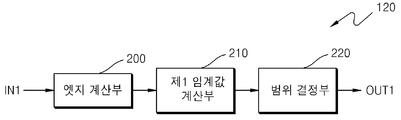••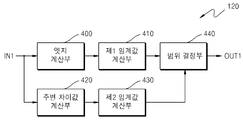•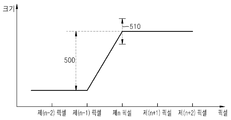••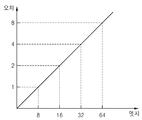•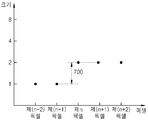•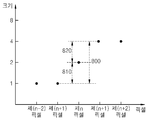•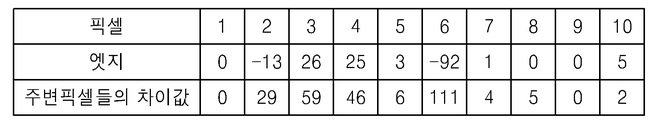•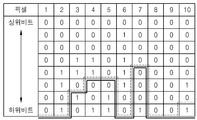•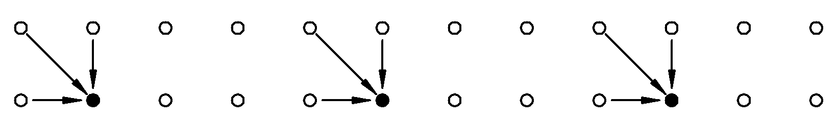•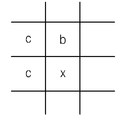•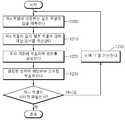•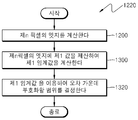•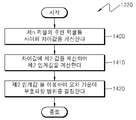•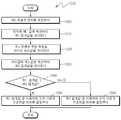## Classifications

• HELECTRICITY
• H04ELECTRIC COMMUNICATION TECHNIQUE
• H04NPICTORIAL COMMUNICATION, e.g. TELEVISION
• H04N19/00Methods or arrangements for coding, decoding, compressing or decompressing digital video signals
• H04N19/10Methods or arrangements for coding, decoding, compressing or decompressing digital video signals using adaptive coding
• H04N19/169Methods or arrangements for coding, decoding, compressing or decompressing digital video signals using adaptive coding characterised by the coding unit, i.e. the structural portion or semantic portion of the video signal being the object or the subject of the adaptive coding
• H04N19/184Methods or arrangements for coding, decoding, compressing or decompressing digital video signals using adaptive coding characterised by the coding unit, i.e. the structural portion or semantic portion of the video signal being the object or the subject of the adaptive coding the unit being bits, e.g. of the compressed video stream
• HELECTRICITY
• H04ELECTRIC COMMUNICATION TECHNIQUE
• H04NPICTORIAL COMMUNICATION, e.g. TELEVISION
• H04N19/00Methods or arrangements for coding, decoding, compressing or decompressing digital video signals
• H04N19/10Methods or arrangements for coding, decoding, compressing or decompressing digital video signals using adaptive coding
• H04N19/102Methods or arrangements for coding, decoding, compressing or decompressing digital video signals using adaptive coding characterised by the element, parameter or selection affected or controlled by the adaptive coding
• H04N19/103Selection of coding mode or of prediction mode
• H04N19/11Selection of coding mode or of prediction mode among a plurality of spatial predictive coding modes
• HELECTRICITY
• H04ELECTRIC COMMUNICATION TECHNIQUE
• H04NPICTORIAL COMMUNICATION, e.g. TELEVISION
• H04N19/00Methods or arrangements for coding, decoding, compressing or decompressing digital video signals
• H04N19/10Methods or arrangements for coding, decoding, compressing or decompressing digital video signals using adaptive coding
• H04N19/102Methods or arrangements for coding, decoding, compressing or decompressing digital video signals using adaptive coding characterised by the element, parameter or selection affected or controlled by the adaptive coding
• H04N19/119Adaptive subdivision aspects, e.g. subdivision of a picture into rectangular or non-rectangular coding blocks
• HELECTRICITY
• H04ELECTRIC COMMUNICATION TECHNIQUE
• H04NPICTORIAL COMMUNICATION, e.g. TELEVISION
• H04N19/00Methods or arrangements for coding, decoding, compressing or decompressing digital video signals
• H04N19/10Methods or arrangements for coding, decoding, compressing or decompressing digital video signals using adaptive coding
• H04N19/102Methods or arrangements for coding, decoding, compressing or decompressing digital video signals using adaptive coding characterised by the element, parameter or selection affected or controlled by the adaptive coding
• H04N19/124Quantisation
• HELECTRICITY
• H04ELECTRIC COMMUNICATION TECHNIQUE
• H04NPICTORIAL COMMUNICATION, e.g. TELEVISION
• H04N19/00Methods or arrangements for coding, decoding, compressing or decompressing digital video signals
• H04N19/10Methods or arrangements for coding, decoding, compressing or decompressing digital video signals using adaptive coding
• H04N19/134Methods or arrangements for coding, decoding, compressing or decompressing digital video signals using adaptive coding characterised by the element, parameter or criterion affecting or controlling the adaptive coding
• HELECTRICITY
• H04ELECTRIC COMMUNICATION TECHNIQUE
• H04NPICTORIAL COMMUNICATION, e.g. TELEVISION
• H04N19/00Methods or arrangements for coding, decoding, compressing or decompressing digital video signals
• H04N19/10Methods or arrangements for coding, decoding, compressing or decompressing digital video signals using adaptive coding
• H04N19/134Methods or arrangements for coding, decoding, compressing or decompressing digital video signals using adaptive coding characterised by the element, parameter or criterion affecting or controlling the adaptive coding
• H04N19/136Incoming video signal characteristics or properties
• H04N19/14Coding unit complexity, e.g. amount of activity or edge presence estimation
• HELECTRICITY
• H04ELECTRIC COMMUNICATION TECHNIQUE
• H04NPICTORIAL COMMUNICATION, e.g. TELEVISION
• H04N19/00Methods or arrangements for coding, decoding, compressing or decompressing digital video signals
• H04N19/10Methods or arrangements for coding, decoding, compressing or decompressing digital video signals using adaptive coding
• H04N19/134Methods or arrangements for coding, decoding, compressing or decompressing digital video signals using adaptive coding characterised by the element, parameter or criterion affecting or controlling the adaptive coding
• H04N19/154Measured or subjectively estimated visual quality after decoding, e.g. measurement of distortion
• HELECTRICITY
• H04ELECTRIC COMMUNICATION TECHNIQUE
• H04NPICTORIAL COMMUNICATION, e.g. TELEVISION
• H04N19/00Methods or arrangements for coding, decoding, compressing or decompressing digital video signals
• H04N19/10Methods or arrangements for coding, decoding, compressing or decompressing digital video signals using adaptive coding
• H04N19/169Methods or arrangements for coding, decoding, compressing or decompressing digital video signals using adaptive coding characterised by the coding unit, i.e. the structural portion or semantic portion of the video signal being the object or the subject of the adaptive coding
• H04N19/182Methods or arrangements for coding, decoding, compressing or decompressing digital video signals using adaptive coding characterised by the coding unit, i.e. the structural portion or semantic portion of the video signal being the object or the subject of the adaptive coding the unit being a pixel
• HELECTRICITY
• H04ELECTRIC COMMUNICATION TECHNIQUE
• H04NPICTORIAL COMMUNICATION, e.g. TELEVISION
• H04N19/00Methods or arrangements for coding, decoding, compressing or decompressing digital video signals
• H04N19/50Methods or arrangements for coding, decoding, compressing or decompressing digital video signals using predictive coding
• H04N19/593Methods or arrangements for coding, decoding, compressing or decompressing digital video signals using predictive coding involving spatial prediction techniques

## Abstract

The present invention relates to an apparatus and a method for encoding an image in consideration of a human sensibility characteristic. The apparatus and method include encoding only a value corresponding to a range that can be perceived by a human sensibility characteristic among error values of predicted pixel values corresponding to each pixel do.

## Description

BACKGROUND OF THE INVENTION 1. Field of the Invention The present invention relates to an image encoding apparatus and a method for encoding an image,

BACKGROUND OF THE INVENTION 1. Field of the Invention The present invention relates to a method and apparatus for encoding an image, and more particularly, to a method and apparatus for encoding an image in consideration of human visual characteristics.

In the audio coding, a masking threshold is set by applying a psycho-acoustic model using the human auditory characteristics, and then different bits are assigned to different frequency regions to be encoded.

In video and / or image coding, there are techniques for coding using human visual characteristics. For example, using the YUV format, or using a quantization matrix in the DCT. First, in the YUV format, compression is performed by allocating a small number of bits for chrominance using the characteristic that human being is vulnerable to chromaticity change. Second, the DCT distinguishes between a low frequency region and a high frequency region, and uses a characteristic that is insensitive to a high frequency region due to the human sensibility characteristic, thereby reducing a bit to which quantization of a high frequency region is largely allocated.

SUMMARY OF THE INVENTION An object of the present invention is to provide an apparatus and a method for encoding only a range that can be perceived in consideration of human visual characteristics.

According to an aspect of the present invention, there is provided a method of encoding an image using a luminance characteristic, the method comprising: calculating an error between a value of a predetermined pixel and a value of a reference pixel corresponding to the predetermined pixel; Determining a range to be encoded among the calculated errors as a predetermined method capable of determining a range in which the sensory characteristics can be recognized; And encoding only the calculated error value corresponding to the determined range.

According to an aspect of the present invention, there is provided a recording medium including: an error calculation unit calculating an error between a value of a predetermined pixel and a value of a reference pixel corresponding to the predetermined pixel among pixels constituting an image; Determining a range to be encoded among the calculated errors as a predetermined method capable of determining a range in which the sensory characteristics can be recognized; And encoding only the calculated error value corresponding to the determined range. The method may be read by a computer recording a program for causing a computer to execute the method.

According to an aspect of the present invention, there is provided an image encoding apparatus using an image sensor, the image encoding apparatus including an error calculation unit for calculating an error between a value of a predetermined pixel and a value of a reference pixel corresponding to the predetermined pixel, ; A coding range determination unit that determines a range to be coded among the calculated errors as a predetermined method that can determine a range in which a sensible characteristic can be recognized; And an encoding unit encoding only the calculated error value corresponding to the determined range.

DETAILED DESCRIPTION OF THE PREFERRED EMBODIMENTS Hereinafter, embodiments of an image encoding apparatus and method using a spectral characteristic according to the present invention will be described in detail with reference to the accompanying drawings.

FIG. 1 is a block diagram of an image encoding apparatus according to an embodiment of the present invention. Referring to FIG. 1, an image encoding apparatus using a spectral characteristic includes a predictor 100, an error calculator 110, (120) and an encoding unit (130).

The prediction unit 100 receives the pixels constituting the image from the input terminal IN and predicts the value of the reference pixel corresponding to each pixel according to a predetermined method. 10 is a conceptual diagram showing an embodiment for predicting the value of a predetermined pixel in the predicting unit 100. In FIG. In FIG. 10, a value of a predetermined pixel can be predicted using values of pixels provided in the upward, leftward, and leftward directions with respect to a predetermined pixel. Can be predicted using an edge detecting method in one embodiment of a predetermined scheme used for predicting the value of the reference pixel in the predicting unit 100. [ With this edge detection method, IEEE Trans. On Consumer Electronics, Vol. 47, No. 3, 2001, pp. 466-476, the method disclosed in "Two low cost algorithms for improved diagonal edge detection in JPEG-LS" can be used. An embodiment for predicting the value of a predetermined pixel 'x' using the edge detection method will be described with reference to FIG. The algorithm of the edge detection method for pixel 'x' is as follows.

if (c > = max (a, b)) P = min (a, b);

else {

if (c < = min (a, b)) P = max (a, b);

else P = a + b-c;

}

Here, 'P' is the value of the reference pixel corresponding to the 'x' pixel, 'a' is the value of the pixel located in the left direction, 'b' is the value of the pixel located in the upward direction, 'c' Lt; RTI ID = 0.0 &gt; direction.

According to this algorithm, the value 'P' of the reference pixel is determined according to whether the edge is horizontal or vertical and what is a large value between 'a' and 'b'. Assuming that 'c' is greater than or equal to the larger of 'a' and 'b', if the smaller of 'a' and 'b' is 'a' The value 'P' is determined as 'a', and if the smaller value of 'a' and 'b' is 'b', the edge has a vertical edge and the reference pixel value 'P' is determined as 'b'. On the other hand, assuming that the smaller value of 'a' and 'b' is greater than or equal to 'c', if the larger of 'a' and 'b' is 'a' The value 'P' is determined as 'a', and when a larger value of 'a' and 'b' is 'b', the edge has a vertical direction and the reference pixel value 'P' is determined as 'b'. Also, assuming that 'c' is larger than a smaller value of 'a' and 'b' and smaller than a larger value of 'a' and 'b', it is difficult to determine the direction of the edge, Is determined by adding 'b' to 'a' and subtracting 'c', considering both 'a', 'b' and 'c'.

The error calculation unit 110 calculates an error between the value of the pixel input from the input terminal IN and the value of the reference pixel predicted corresponding to each pixel in the predictor 100.

The coding range determination unit 120 determines a range to be coded among the errors calculated by the error calculation unit 110. In determining the range by the coding range determination unit 120, a predetermined method capable of determining a range that can be perceived by the human sensibility characteristic is used. In other words, the coding range determination unit 120 determines a coding range corresponding to a value corresponding to an upper bit that can be recognized on the human sensibility characteristic among the errors calculated by the error calculation unit 110 and a value corresponding to a lower bit Distinguish the values.

Examples of the coding range determination unit 120 are shown in Figs. 2, 3, and 4. Fig.

FIG. 2 is a block diagram of an embodiment for determining a range to be coded based on an error calculated by the error calculator 110 using an edge of a predetermined pixel. The coding range determiner 120 includes an edge calculator 200 A first threshold value calculation unit 210, and a range determination unit 220.

The edge calculation unit 200 calculates an edge of each pixel. Here, the edge refers to a difference value between a value of a predetermined pixel and a value of a peripheral pixel provided in the periphery of the predetermined pixel. 7, the edge of the n-th pixel is the difference value of the n-th pixel with respect to the value of the (n-1) pixel, and corresponds to the interval shown in the identification number 700.

The first threshold value calculation unit 210 calculates a first threshold value by dividing a predetermined first value by the edge of each pixel calculated by the edge calculation unit 200.

The range determination unit 220 determines a range to be coded based on the error calculated by the error calculation unit 110 using the first threshold value calculated by the first threshold value calculation unit 210. There are two embodiments described below in which the range determination unit 220 determines a range to be coded by using the first threshold value.

First, a range in which only the values not included in the first threshold value among the errors calculated by the error calculation unit 110 are encoded is determined. 5A, the edge calculation unit 200 calculates the interval corresponding to the identification number 500 at the edge of the n-th pixel, and in the first threshold value calculation unit 210, When '8' is previously set as a value for dividing the first threshold value, the range determination unit 220 calculates the first threshold value by dividing '8' in the interval corresponding to the identification number 500, A range corresponding to the identification number 510 that allows an error corresponding to the first threshold value is determined.

Assume that the fourth pixel shown in FIG. 9A, for example, the edge calculator 200 calculates '25' as the edge of the fourth pixel. However, in the embodiment of FIGS. 9A and 9B, it is assumed that the edge and the error are the same since it is common to use the neighboring pixels in predicting the reference pixel in the predicting unit 100. FIG. The first threshold value calculation unit 210 calculates a first threshold value '3.125' by dividing the first value '8', which is a predetermined value, to '25', which is the edge of the fourth pixel, and the range determination unit 220 The lower 2 bits corresponding to the first threshold value of '3.125' or less are determined as a range not to be encoded, and the upper 3 bits corresponding to the first threshold value '3.125' or more are determined as the coding range. Accordingly, the range determination unit 220 determines a range for encoding only the upper three bits '110' among '11001' corresponding to the error '25' of the fourth pixel, and does not encode the lower two bits '01' .

Second, a range not to be included in the first threshold value among the errors calculated by the error calculation unit 110 and an error value that is not encoded in the encoder are determined in consideration of an algorithm for decoding in the decoder. An error value that is not encoded in the encoder is restored by a predetermined algorithm in the decoder. As an example of the predetermined algorithm, it is possible to restore at every possible number of expected values, average values, and intermediate values within a range of error values not encoded by the encoder, and to restore values corresponding to each error value to a table And can be restored by reading and storing. If the encoder decodes the decoded data into all possible intermediate values within the range of the uncoded error value, the lower bit to be uncoded, which is determined by the first threshold value, can be increased by one bit. Therefore, The determined upper bit to be encoded can be reduced by 1 bit.

For example, in the case of the fourth pixel shown in FIG. 9A, which is the example described in the first method, the range determination unit 220, based on the first threshold value '3.125' calculated by the first threshold value calculation unit 210, The lower 2 bits corresponding to the first threshold value of '3.125' or less are determined as a range not to be encoded, and the upper 3 bits corresponding to the first threshold value '3.125' or more are determined as the coding range. Accordingly, the range determination unit 220 determines a range for encoding only the upper three bits '110' among '11001' corresponding to the error '25' of the fourth pixel, and does not encode the lower two bits '01' .

However, according to the second scheme, when decoding is performed at the decoding end with all possible intermediate values within the range of error values not encoded in the encoder, one bit of the upper bits is not further encoded but only the upper two bits '11' And the lower 3 bits are determined as a range not to be encoded. In this case, too, the lower 3 bits are recovered from the decoder to '100' which is an intermediate value of '000' to '111' which can be represented by lower 3 bits not transmitted by the encoder. And finally restored to '11100'. The reconstructed '11100' is included in the first threshold since there is no difference between '11001' and '11', which are errors of the fourth pixel. Therefore, even though one bit among the upper bits determined as the first threshold value is determined as a range for encoding less, the decoder can not recognize the human sensibility because it is restored to the value of the pixel within the threshold value range in the decoder.

In the same manner, by calculating the first threshold value with the edges for the first through third pixels and the fifth through tenth pixels shown in FIG. 9A, bits corresponding to higher bits than the dotted line are encoded Can be determined as the range to do. Since the embodiment of FIGS. 9A and 9B assumes that the first value is set to '8' in advance, it can be determined that only the upper two bits are to be encoded.

In other words, if the error of the reference pixel and the edge of a certain pixel are the same and a preset value for dividing the first threshold is 8, the range is determined to be the range of encoding only the upper 3 bits when implemented by the first method, Scheme, when the decoder decodes lower bits that are not encoded by the encoder, an algorithm of a method of adding an intermediate value is used. However, the present invention is not limited to the preset value '8', but may be performed by setting other values according to the operating environment such as display characteristics.

FIG. 3 is a block diagram of an embodiment for determining a range to be coded based on errors calculated by the error calculator 110 using surrounding pixels provided around a predetermined pixel. The coding range determiner 120 determines A difference value calculation unit 300, a second threshold value calculation unit 310, and a range determination unit 320.

The neighboring difference value calculation unit 300 calculates a difference value between neighboring pixels provided around each pixel. 8, the difference value between neighboring pixels of the n-th pixel refers to a difference value between the (n-1) -th pixel and the (n + 1) -th pixel provided in the periphery of the n-th pixel, Corresponds to the interval shown in the identification number 800.

The second threshold value calculation unit 310 calculates a second threshold value by dividing a predetermined second value by a difference value between adjacent pixels of each pixel calculated by the surrounding difference value calculation unit 300. [

Here, the preset value may be different from the first value described in FIG. The difference value between neighboring pixels provided in the vicinity of a specific pixel corresponds to a value obtained by multiplying the maximum difference value between the specific pixel and the neighboring pixel by &quot; 2 &quot;. Referring to FIG. 8, the neighboring pixels of the n-th pixel include (n-1) th and (n + 1) th pixels. The first edge, which is the difference between the (n-1) th pixel and the (n + 1) th pixel, is a value corresponding to the identification number 810, The second edge, which is the difference value, corresponds to the identification number 820. These relations are expressed in the following equations.

[Equation 1]

Here, "edge1" is the first edge, "edge2" is the second edge, and "neighbor"

Since the difference value between the edge and the neighboring pixels has the relationship as expressed by Equation 1, it is assumed that the edge is equal to the difference value between neighboring pixels, and the value obtained by dividing the first value by 2 is set as the second value .

The range determining unit 320 determines a range to be coded based on the error calculated by the error calculating unit 110 using the second threshold value calculated by the second threshold calculating unit 310. There are two embodiments described below in which the range determination unit 320 determines the range to be encoded using the second threshold value.

First, it is determined that only a value that is not included in the second threshold among the errors calculated by the error calculating unit 110 is encoded. 5B, the neighboring difference value calculating unit 300 calculates the difference value between the (n-1) th pixel and the (n + 1) th pixel corresponding to neighboring pixels of the n-th pixel, The second threshold value calculation unit 310 divides the interval corresponding to the identification number 520 by '16' if '16' is set as the second value for dividing the second threshold value And the range determination unit 320 permits the error included in the second threshold value for the value of the n-th pixel.

Assuming that the fourth pixel shown in FIG. 9A, for example, '46' is calculated as the difference value of the neighboring pixels of the fourth pixel in the neighboring difference calculating unit 300, will be described. However, in the embodiment of FIGS. 9A and 9B, it is assumed that the edge and the error are the same since it is common to use the neighboring pixels in predicting the reference pixel in the predicting unit 100. FIG. The second threshold value calculation unit 310 calculates a second threshold value '2.875' by dividing the difference value '46' of the neighboring pixels of the fourth pixel by '16', and the range determination unit 320 2.875 &quot; and the upper 3 bits corresponding to the second threshold value &quot; 2.875 &quot; or more are determined as the coding range. Accordingly, the range determination unit 320 determines a range for encoding only the upper three bits '110' among '11001' corresponding to the error '25' of the fourth pixel, and does not encode the lower two bits '01' .

Second, a range that is not included in the second threshold value among the errors calculated by the error calculation unit 110 and an error value that is not encoded in the encoder are determined in consideration of an algorithm for decoding in the decoder. An error value that is not encoded in the encoder is restored by a predetermined algorithm in the decoder. As an example of the predetermined algorithm, it is possible to restore at every possible number of expected values, average values, and intermediate values within a range of error values not encoded by the encoder, and to restore values corresponding to each error value to a table And can be restored by reading and storing. If the decoder restores the decoded data with all the possible intermediate values within the range of the uncoded error value, the lower bit to be uncoded, which is determined by the second threshold value, can be increased by 2 bits. The determined upper bit to be encoded can be reduced by 1 bit. In this way, by calculating the second threshold value as the difference value of the neighboring pixels for the first through third pixels and the fifth through tenth pixels shown in FIG. 9A, The corresponding bit can be determined as a range to be encoded. Since the embodiment of FIGS. 9A and 9B assumes that the second value is set to '16' by default, it can be determined that only the upper 3 bits are to be coded.

FIG. 4 is a block diagram of an embodiment for determining a range to be coded based on the error calculated by the error calculator 110 using the edge of a predetermined pixel or the difference between neighboring pixels provided around the predetermined pixel. The range determination unit 120 includes an edge calculation unit 400, a first threshold value calculation unit 410, a peripheral difference value calculation unit 420, a second threshold value calculation unit 430 and a range determination unit 440 .

The edge calculation unit 400 calculates an edge of each pixel. Here, the edge refers to a difference value between a value of a predetermined pixel and a value of a peripheral pixel provided in the periphery of the predetermined pixel. 7, the edge of the n-th pixel is the difference value of the n-th pixel with respect to the value of the (n-1) pixel, and corresponds to the interval shown in the identification number 700.

The first threshold value calculation unit 410 calculates a first threshold value by dividing a predetermined first value by the edge of each pixel calculated by the edge calculation unit 400. [

The peripheral difference value calculation unit 420 calculates a difference value between neighboring pixels provided around each pixel. 8, the difference value between neighboring pixels of the n-th pixel refers to a difference value between the (n-1) -th pixel and the (n + 1) -th pixel provided in the periphery of the n-th pixel, Corresponds to the interval shown in the identification number 800.

The second threshold value calculation unit 430 calculates a second threshold value by dividing a predetermined second value by a difference value between adjacent pixels of each pixel calculated by the surrounding difference value calculation unit 420. [ Here, the preset value may be different from the first value described in FIG. The difference value between neighboring pixels provided in the vicinity of a specific pixel corresponds to a value obtained by multiplying the maximum difference value between the specific pixel and the neighboring pixel by &quot; 2 &quot;. Referring to FIG. 8, the neighboring pixels of the n-th pixel include (n-1) th and (n + 1) th pixels. The first edge, which is the difference between the (n-1) th pixel and the (n + 1) th pixel, is a value corresponding to the identification number 810, The second edge, which is the difference value, corresponds to the identification number 820. These relations are expressed in the following equations.

&Quot; (2) &quot;

Here, "edge1" is the first edge, "edge2" is the second edge, and "neighbor"

Since the difference value between the edge and the neighboring pixels has the relationship as shown in Equation 2, it is assumed that the edge is equal to the difference value between neighboring pixels, and the value obtained by dividing the first value by 2 is set as the second value .

The range determination unit 440 selects the largest threshold value among the first threshold value calculated by the first threshold value calculation unit 410 and the second threshold value calculated by the second threshold value calculation unit 430, A range to be coded is determined based on the error calculated by the error calculator 110 using a large threshold value. If the first threshold value is larger than the second threshold value, a range to be encoded is determined based on the error calculated by the error calculating unit 110 in the same manner as the range determining unit 220 shown in FIG. 2, If the value is larger than the first threshold value, the range to be encoded is determined based on the error calculated by the error calculating unit 110 in the same manner as the range determining unit 320 shown in FIG.

9A and 9B are tables showing an embodiment for determining the range in the range determination unit 440. In FIG. FIG. 9A is a table showing the differences between the edges of the first to tenth pixels and the neighboring pixels of the first to tenth pixels, and FIG. 9B is a diagram illustrating errors of the first to tenth pixels from the upper bit to the lower bit And a table expressed in bit-plane units. However, in the embodiment of FIGS. 9A and 9B, it is assumed that the edge and the error are the same since it is common to use the neighboring pixels in predicting the reference pixel in the predicting unit 100. FIG.

The first threshold value calculated by the first threshold value calculation unit 410 is indicated by a dashed line in FIG. 9B, and the second threshold value calculated by the second threshold value calculation unit 430 is indicated by a solid line in FIG. 9B have. The range determination unit 440 determines a larger value among the dotted line and the solid line as the threshold value and determines the range to encode the bit-plane of the error provided in the upper bit than the determined threshold value.

1, the encoding unit 130 encodes only the error calculated by the error calculation unit 110 corresponding to the range determined by the encoding range determination unit 120. [ More specifically, the encoding unit 130 encodes only the error calculated by the error calculation unit 110, which corresponds to the upper bit determined to be encoded by the encoding range determination unit 120, to generate a bit stream, Lt; / RTI &gt;

FIG. 12 is a flowchart illustrating an image encoding method using a spectral characteristic according to the present invention.

First, the pixels constituting the image are input and a value of a reference pixel corresponding to the n-th pixel is predicted according to a predetermined method (operation 1200). FIG. 10 is a conceptual diagram illustrating an embodiment for predicting a value of a predetermined pixel in operation 1200. FIG. In FIG. 10, a value of a predetermined pixel can be predicted using values of pixels provided in the upward, leftward, and leftward directions with respect to a predetermined pixel. May be predicted using an edge detecting method in one embodiment of a predetermined scheme used in step 1200 to predict the value of the reference pixel. With this edge detection method, IEEE Trans. On Consumer Electronics, Vol. 47, No. 3, 2001, pp. 466-476, the method disclosed in "Two low cost algorithms for improved diagonal edge detection in JPEG-LS" can be used. An embodiment for predicting the value of a predetermined pixel 'x' using the edge detection method will be described with reference to FIG. The algorithm of the edge detection method for pixel 'x' is as follows.

if (c > = max (a, b)) P = min (a, b);

else {

if (c < = min (a, b)) P = max (a, b);

else P = a + b-c;

}

Here, 'P' is the value of the reference pixel corresponding to the 'x' pixel, 'a' is the value of the pixel located in the left direction, 'b' is the value of the pixel located in the upward direction, 'c' Lt; RTI ID = 0.0 &gt; direction.

According to this algorithm, the value 'P' of the reference pixel is determined according to whether the edge is horizontal or vertical and what is a large value between 'a' and 'b'. Assuming that 'c' is greater than or equal to the larger of 'a' and 'b', if the smaller of 'a' and 'b' is 'a' The value 'P' is determined as 'a', and if the smaller value of 'a' and 'b' is 'b', the edge has a vertical edge and the reference pixel value 'P' is determined as 'b'. On the other hand, assuming that the smaller value of 'a' and 'b' is greater than or equal to 'c', if the larger of 'a' and 'b' is 'a' The value 'P' is determined as 'a', and when a larger value of 'a' and 'b' is 'b', the edge has a vertical direction and the reference pixel value 'P' is determined as 'b'. Also, assuming that 'c' is larger than a smaller value of 'a' and 'b' and smaller than a larger value of 'a' and 'b', it is difficult to determine the direction of the edge, Is determined by adding 'b' to 'a' and subtracting 'c', considering both 'a', 'b' and 'c'.

In operation 1200, an error between the predicted reference pixel value and the n-th pixel value corresponding to the n-th pixel is calculated (operation 1210).

In operation 1220, a range to be coded is determined based on the error of the n-th pixel calculated in operation 1210. In the determination of the range in operation 1220, a predetermined method that can determine a range that can be perceived by the human sensibility characteristic is used. In other words, in operation 1220, a value corresponding to an upper bit that can be recognized on a human's sensory characteristic and a value corresponding to a lower bit that can not be recognized on a human's sensory characteristic are distinguished from errors calculated in operation 1210.

13, 14, and 15 are examples of this step 1220.

FIG. 13 is a flowchart illustrating an embodiment of determining a range to be coded based on the error of the n-th pixel calculated in operation 1210 using the edge of the n-th pixel.

First, the edge of the n-th pixel is calculated (operation 1300). Here, the edge refers to a difference value between a value of a predetermined pixel and a value of a peripheral pixel provided in the periphery of the predetermined pixel. 7, the edge of the n-th pixel is the difference value of the n-th pixel with respect to the value of the (n-1) pixel, and corresponds to the interval shown in the identification number 700.

In operation 1310, the first threshold value is calculated by dividing the first value preset in the edge of the n-th pixel calculated in operation 1300.

In operation 1320, a range to encode the error of the n-th pixel calculated in operation 1210 is determined using the first threshold value of the n-th pixel calculated in operation 1310. In step 1320, there are two embodiments described below that determine the range to be coded in the error using the first threshold value.

First, a range in which only the values not included in the first threshold value among the errors calculated in operation 1210 is encoded is determined. Referring to FIG. 5A, in step 1300, the interval corresponding to the identification number 500 is calculated as the edge of the n-th pixel. In step 1310, a value for dividing the first threshold value 8 'is set to' 1 ', the first threshold value is calculated by dividing' 8 'in the interval corresponding to the identification number 500. In step 1320, an error corresponding to the first threshold value is applied to the value of the n- The range corresponding to the allowable identification number 510 is determined.

Assume that the fourth pixel shown in FIG. 9A, for example, in step 1300, '25' is calculated as the edge of the fourth pixel. However, in the embodiment of FIGS. 9A and 9B, since it is common to use neighboring pixels in predicting the reference pixel in operation 1200, it is assumed that the edge and the error are equal to each other. In step 1310, the first threshold value '3.125' is calculated by dividing the first value '8', which is a predetermined value, to '25', which is the edge of the fourth pixel. In step 1320, Is determined to be a range that does not encode the lower 2 bits corresponding to the first threshold value, and the upper 3 bits corresponding to the first threshold value of 3.125 or more are encoded. Accordingly, in step 1320, only the upper three bits '110' of '11001' corresponding to the error '25' of the fourth pixel are determined as a range to be encoded, and the lower two bits '01' do.

Second, a range to be included in the first threshold value among the errors calculated in operation 1210 and an error value not encoded in the encoder are determined in consideration of an algorithm for decoding in the decoder. The error value that is not encoded in the encoder is restored by a predetermined algorithm in the decoder. As an example of the predetermined algorithm, it is possible to restore at every possible number of expected values, average values, and intermediate values within a range of error values not encoded by the encoder, and to restore values corresponding to each error value to a table And can be restored by reading and storing. If the encoder decodes the decoded data into all possible intermediate values within the range of the uncoded error value, the lower bit to be uncoded, which is determined by the first threshold value, can be increased by one bit. Therefore, The determined upper bit to be encoded can be reduced by 1 bit.

For example, in the case of the fourth pixel shown in FIG. 9A, which is the example described in the first method, the first threshold value '3.125' calculated in step 1310 is used as a reference, and in step 1320, the first threshold value '3.125' Is determined to be a range that does not encode the lower 2 bits corresponding to the first threshold value, and the upper 3 bits corresponding to the first threshold value of 3.125 or more are encoded. Accordingly, in step 1320, only the upper three bits '110' of '11001' corresponding to the error '25' of the fourth pixel are determined as a range to be encoded, and the lower two bits '01' do.

However, according to the second scheme, when decoding is performed at the decoding end with all possible intermediate values within the range of error values not encoded in the encoder, one bit of the upper bits is not further encoded but only the upper two bits '11' And the lower 3 bits are determined as a range not to be encoded. In this case, too, the lower 3 bits are recovered from the decoders to '100', which is an intermediate value of '000' to '111' which can be expressed by the lower 3 bits not transmitted by the encoder, And finally restored to '11100'. The reconstructed '11100' is included in the first threshold since there is no difference between '11001' and '11', which are errors of the fourth pixel. Therefore, even though one bit among the upper bits determined as the first threshold value is determined as a range for encoding less, the decoder can not recognize the human sensibility because it is restored to the value of the pixel within the threshold value range in the decoder.

In the same manner, by calculating the first threshold value with the edges for the first through third pixels and the fifth through tenth pixels shown in FIG. 9A, bits corresponding to higher bits than the dotted line are encoded Can be determined as the range to do. Since the embodiment of FIGS. 9A and 9B assumes that the first value is set to '8' in advance, it can be determined that only the upper two bits are to be encoded.

In other words, if the error of the reference pixel and the edge of a certain pixel are the same and a preset value for dividing the first threshold is 8, the range is determined to be the range of encoding only the upper 3 bits when implemented by the first method, Scheme, when the decoder decodes lower bits that are not encoded by the encoder, an algorithm of a method of adding an intermediate value is used. However, the present invention is not limited to the preset value '8', but may be performed by setting other values according to the operating environment such as display characteristics.

FIG. 14 is a flowchart illustrating an example of determining a range to be coded based on the error calculated in operation 1210 using neighboring pixels provided around the n-th pixel.

First, a difference value between neighboring pixels provided around the nth pixel is calculated (operation 1400). 8, the difference value between neighboring pixels of the n-th pixel refers to a difference value between the (n-1) -th pixel and the (n + 1) -th pixel provided in the periphery of the n-th pixel, Corresponds to the interval shown in the identification number 800.

In operation 1410, the second threshold value is calculated by dividing the difference between adjacent pixels of each pixel calculated in operation 1400 by a predetermined second value.

Here, the preset value may be different from the first value described in FIG. The difference value between neighboring pixels provided in the vicinity of a specific pixel corresponds to a value obtained by multiplying the maximum difference value between the specific pixel and the neighboring pixel by &quot; 2 &quot;. Referring to FIG. 8, the neighboring pixels of the n-th pixel include (n-1) th and (n + 1) th pixels. The first edge, which is the difference between the (n-1) th pixel and the (n + 1) th pixel, is a value corresponding to the identification number 810, The second edge, which is the difference value, corresponds to the identification number 820. These relations are expressed in the following equations.

&Quot; (3) &quot;

Here, "edge1" is the first edge, "edge2" is the second edge, and "neighbor"

Since the difference value between the edge and the neighboring pixels has the relationship as expressed by Equation 1, it is assumed that the edge is equal to the difference value between neighboring pixels, and the value obtained by dividing the first value by 2 is set as the second value .

In operation 1420, a range to be coded is calculated using the second threshold calculated in operation 1410 and the error is calculated in operation 1210. In step 1420, there are two embodiments described below that determine the range to be coded in the error using the second threshold value.

First, a range in which only values that are not included in the second threshold value among the errors calculated in operation 1410 are encoded is determined. Referring to FIG. 5B, in operation 1400, an interval corresponding to the identification number 520, which is a difference between the (n-1) th pixel and the (n + 1) th pixel corresponding to the neighboring pixels of the n- If '16' is set as a second value for dividing the second threshold value in operation 1410, a second threshold value is calculated by dividing '16' in the interval corresponding to the identification number 520. In operation 1420, An error included in the second threshold value for the value of the n-th pixel is allowed.

For example, assuming that '46' is calculated as the difference value of the neighboring pixels of the fourth pixel in step 1400, the fourth pixel shown in FIG. 9A will be described. However, in the embodiment of FIGS. 9A and 9B, since it is common to use neighboring pixels in predicting the reference pixel in operation 1200, it is assumed that the edge and the error are equal to each other. In step 1410, the second threshold value '2.875' is calculated by dividing '16' to '46', which is the difference value of the neighboring pixels of the fourth pixel. In step 1420, the second threshold value is 2.875 or less And decides the range of encoding the upper 3 bits corresponding to the second threshold value of 2.875 or more. Accordingly, in step 1420, only the upper three bits '110' of '11001' corresponding to the error '25' of the fourth pixel are determined to be a range for encoding, and the lower two bits '01' do.

Second, a range not to be included in the second threshold value among the errors calculated in operation 1210 and an error value not encoded in the encoder are determined in consideration of an algorithm for decoding in the decoder. An error value that is not encoded in the encoder is restored by a predetermined algorithm in the decoder. As an example of the predetermined algorithm, it is possible to restore at every possible number of expected values, average values, and intermediate values within a range of error values not encoded by the encoder, and to restore values corresponding to each error value to a table And can be restored by reading and storing. If the decoder restores the decoded data to all possible intermediate values within the error value range that is not encoded by the encoder, it is possible to increase the lower-order bits to be uncoded, which are determined by the second threshold value, by two bits. The determined upper bit to be encoded can be reduced by 1 bit. In this way, by calculating the second threshold value as the difference value of the neighboring pixels for the first through third pixels and the fifth through tenth pixels shown in FIG. 9A, The corresponding bit can be determined as a range to be encoded. Since the embodiment of FIGS. 9A and 9B assumes that the second value is set to '16' by default, it can be determined that only the upper 3 bits are to be coded.

FIG. 15 is a flowchart illustrating an embodiment of determining a range to be coded based on an error calculated in operation 1210 using difference values of neighboring pixels provided around an n-th pixel or an n-th pixel.

First, the edge of the n-th pixel is calculated (operation 1500). Here, the edge refers to a difference value between a value of a predetermined pixel and a value of a peripheral pixel provided in the periphery of the predetermined pixel. 7, the edge of the n-th pixel is the difference value of the n-th pixel with respect to the value of the (n-1) pixel, and corresponds to the interval shown in the identification number 700.

The first threshold value is calculated by dividing the first value preset in the edge of the n-th pixel calculated in operation 1500 (operation 1510).

A difference value between neighboring pixels provided around the nth pixel is calculated (operation 1520). 8, the difference value between neighboring pixels of the n-th pixel refers to a difference value between the (n-1) -th pixel and the (n + 1) -th pixel provided in the periphery of the n-th pixel, Corresponds to the interval shown in the identification number 800.

In operation 1530, the second threshold value is calculated by dividing the difference value between neighboring pixels of each pixel calculated in operation 1520 by a predetermined value.

Here, the preset value may be different from the first value described in FIG. The difference value between neighboring pixels provided in the vicinity of a specific pixel corresponds to a value obtained by multiplying the maximum difference value between the specific pixel and the neighboring pixel by &quot; 2 &quot;. Referring to FIG. 8, the neighboring pixels of the n-th pixel include (n-1) th and (n + 1) th pixels. The first edge, which is the difference between the (n-1) th pixel and the (n + 1) th pixel, is a value corresponding to the identification number 810, The second edge, which is the difference value, corresponds to the identification number 820. These relations are expressed in the following equations.

&Quot; (4) &quot;

Here, "edge1" is the first edge, "edge2" is the second edge, and "neighbor"

Since the difference value between the edge and the neighboring pixels has the relationship as shown in Equation 2, it is assumed that the edge is equal to the difference value between neighboring pixels, and the value obtained by dividing the first value by 2 is set as the second value .

In operation 1540, it is determined whether any of the first threshold value calculated in operation 1510 and the second threshold value calculated in operation 1530 is larger.

If it is determined in operation 1540 that the first threshold value is greater than the second threshold value, a range to be encoded is determined based on the first threshold value calculated in operation 1510 ). In operation 1550, the same operation as in the operation 1320 of FIG. 13 may be performed.

If it is determined in operation 1540 that the second threshold value is larger than the first threshold value, a range to be encoded is determined based on the second threshold value calculated in Operation 1510 ). In operation 1560, the same operation as in operation 1420 of FIG. 14 may be performed.

FIGS. 9A and 9B are tables illustrating embodiments for determining ranges in steps 1540 to 1560. FIG. FIG. 9A is a table showing the differences between the edges of the first to tenth pixels and the neighboring pixels of the first to tenth pixels, and FIG. 9B is a diagram illustrating errors of the first to tenth pixels from the upper bit to the lower bit And a table expressed in bit-plane units. However, in the embodiment of FIGS. 9A and 9B, it is assumed that the edge and the error are the same since it is common to use the neighboring pixels in predicting the reference pixel in the predicting unit 100. FIG.

The first threshold value calculated in operation 1510 is indicated by a dashed line in FIG. 9B, and the second threshold value calculated in operation 1530 is indicated by a solid line in FIG. 9B. In steps 1540 to 1560, a larger value among the dotted line and the solid line is determined as a threshold value, and a range for coding the bit-plane of the error provided in the upper bit than the determined threshold value is determined.

Referring again to FIG. 12, in operation 1230, only the error of the n-th pixel calculated in operation 1230 corresponding to the range determined in operation 1220 is encoded. More specifically, in operation 1230, only the error calculated in operation 1210 corresponding to the upper bits determined to be encoded in operation 1220 is encoded.

After operation 1230, it is determined whether the n-th pixel is the last pixel to be encoded (operation 1240).

If it is determined that the n-th pixel is not the last pixel to be encoded, '1' is added to 'n', and steps 1200 to 1230 are repeated.

While the present invention has been particularly shown and described with reference to exemplary embodiments thereof, it is to be understood that the invention is not limited to the disclosed embodiments, but, on the contrary, is intended to cover various modifications and equivalent arrangements included within the spirit and scope of the appended claims. . Accordingly, the true scope of the present invention should be determined by the appended claims.

Furthermore, the present invention can be embodied as a computer-readable code on a computer-readable recording medium (including all devices having an information processing function). A computer-readable recording medium includes all kinds of recording apparatuses in which data that can be read by a computer system is stored. Examples of computer-readable recording devices include ROM, RAM, CD-ROM, magnetic tape, floppy disk, optical data storage, and the like.

FIG. 1 is a block diagram of an embodiment of an image encoding apparatus using a spectral characteristic according to the present invention.

FIG. 2 is a block diagram of an embodiment for determining a range to be coded using the error calculated by the error calculator 110 using the edge of a predetermined pixel.

FIG. 3 is a block diagram of an embodiment for determining a range to be coded based on errors calculated by the error calculator 110 using surrounding pixels provided around a predetermined pixel.

FIG. 4 is a block diagram of an embodiment for determining a range to be coded based on the error calculated by the error calculator 110 using the edge of a predetermined pixel or the difference value of neighboring pixels provided around the predetermined pixel.

5A is a graph for explaining the error range of the n-th pixel calculated using the edge of the n-th pixel.

FIG. 5B is a graph illustrating a range of an error of an n-th pixel calculated using a difference value of neighboring pixels of an n-th pixel.

6 is a graph for explaining a relationship between an edge and an error with a threshold value being a slope.

Fig. 7 shows a graph for explaining the edge of the n-th pixel.

8 is a graph for explaining difference values of neighboring pixels of the n-th pixel.

FIG. 9A is a table showing differences between the edges of the first to tenth pixels and the neighboring pixels of the first to tenth pixels.

FIG. 9B shows a table in which the errors of the first to tenth pixels are expressed in bit-plane units in order from upper bit to lower bit.

FIG. 10 is a conceptual diagram illustrating an embodiment for predicting a value of a predetermined pixel in operation 1200. FIG.

11 is a conceptual diagram for explaining an embodiment for predicting a value of a predetermined pixel 'x'.

FIG. 12 is a flowchart illustrating an image encoding method using a spectral characteristic according to the present invention.

FIG. 13 is a flowchart illustrating an embodiment of determining a range to be coded based on the error of the n-th pixel calculated in operation 1210 using the edge of the n-th pixel.

FIG. 14 is a flowchart illustrating an embodiment of determining a range to be coded based on the error calculated in operation 1210 using neighboring pixels provided around the n-th pixel.

FIG. 15 is a flowchart illustrating an embodiment of determining a range to be coded based on an error calculated in operation 1210, using difference values of neighboring pixels provided around an edge of an n-th pixel or an n-th pixel.

BRIEF DESCRIPTION OF THE DRAWINGS FIG.

100: prediction unit 110: error calculation unit

120: encoding range determination unit 130: encoding unit

## Claims (17)

1. Calculating an error with respect to a value of a reference pixel corresponding to the predetermined pixel, which is determined based on a value of a predetermined pixel, which is one of pixels constituting an image, and a value of a neighboring pixel provided in the periphery of the predetermined pixel;
Determining a range to be coded among the calculated errors in a predetermined manner; And
And encoding only the range corresponding to the determined range from the calculated error value.
2. 2. The method of claim 1, wherein the determining comprises:
Calculating a difference value between the value of the predetermined pixel and a value of a peripheral pixel provided in the periphery of the predetermined pixel; And
And determining a range to be coded based on the calculated error using the calculated difference value.
3. 3. The method of claim 2, wherein determining
Calculating a threshold value by dividing a predetermined value by the calculated difference value;
Determining an upper bit number that can represent only the calculated error value corresponding to the calculated threshold value or more,
The encoding step
And coding only the range corresponding to the determined upper bit number in the calculated error value.
4. 2. The method of claim 1, wherein the determining comprises:
Calculating a difference value between neighboring pixels provided around the predetermined pixel; And
And determining a range to be coded based on the calculated error using the calculated difference value.
5. 5. The method of claim 4, wherein determining
Calculating a threshold value by dividing a predetermined value by the calculated difference value;
Determining an upper bit number that can represent only the calculated error value corresponding to the calculated threshold value or more,
The encoding step
And coding only the range corresponding to the determined upper bit number in the calculated error value.
6. 2. The method of claim 1, wherein the determining comprises:
Calculating a difference value between the value of the predetermined pixel and a value of a neighboring pixel provided in the periphery of the predetermined pixel or a difference value between the neighboring pixels; And
And determining a range to be coded based on the calculated error using the calculated difference value.
7. 7. The method of claim 6, wherein determining
Calculating a first difference value between the value of the predetermined pixel and the value of the surrounding pixel;
Calculating a second difference value between the neighboring pixels;
Calculating a first threshold value by dividing a value previously set in the first difference value, and calculating a second threshold value by dividing a predetermined value by a second difference value; And
Determining a number of upper bits that can represent only the calculated error value that is greater than or equal to a larger value of the first threshold value and the second threshold value,
The encoding step
And coding only the range corresponding to the determined upper bit number in the calculated error value.
8. The method of claim 1, wherein the calculated error value not corresponding to the determined range is reconstructed by the decoder in any one of an expected value, an average value, and an intermediate value.
9. Calculating an error with respect to a value of a reference pixel corresponding to the predetermined pixel, which is determined based on a value of a predetermined pixel, which is one of pixels constituting an image, and a value of a neighboring pixel provided in the periphery of the predetermined pixel;
Determining a range to be coded among the calculated errors in a predetermined manner; And
And encoding only the range corresponding to the determined range from the calculated error value. The computer-readable recording medium according to claim 1,
10. An error calculation for calculating an error with respect to a value of a reference pixel corresponding to the predetermined pixel, which is determined based on a value of a predetermined pixel which is one of pixels constituting the image and a value of a peripheral pixel provided in the periphery of the predetermined pixel part;
An encoding range determination unit for determining a range to be encoded in the calculated error in a predetermined manner; And
And a coding unit for coding only the range corresponding to the determined range from the calculated error value.
11. 11. The apparatus of claim 10, wherein the coding range determination unit
An edge calculating unit for calculating a difference value between the value of the predetermined pixel and a value of a peripheral pixel provided in the periphery of the predetermined pixel; And
And a determination unit determining a range to be coded based on the calculated error using the calculated difference value.
12. 12. The apparatus of claim 11, wherein the coding range determination unit
A threshold value calculation unit for calculating a threshold value by dividing a predetermined value by the calculated difference value;
And a range determination unit for determining an upper bit number that can represent only the calculated error value corresponding to the calculated threshold value or more,
The encoding unit
And coding only the range corresponding to the determined upper bit number in the calculated error value.
13. 11. The apparatus of claim 10, wherein the coding range determination unit
A peripheral difference value calculating unit for calculating a difference value between neighboring pixels provided around the predetermined pixel; And
And a determination unit determining a range to be coded based on the calculated error using the calculated difference value.
14. 14. The apparatus of claim 13, wherein the determining unit
A threshold value calculation unit for calculating a threshold value by dividing a predetermined value by the calculated difference value;
And a range determination unit for determining an upper bit number that can represent only the calculated error value corresponding to the calculated threshold value or more,
The encoding unit
And coding only the range corresponding to the determined upper bit number in the calculated error value.
15. 11. The apparatus of claim 10, wherein the coding range determination unit
A calculation unit for calculating a difference value between the value of the predetermined pixel and a value of neighboring pixels provided around the predetermined pixel or a difference value between the adjacent pixels; And
And a determination unit determining a range to be coded based on the calculated error using the calculated difference value.
16. 16. The apparatus of claim 15, wherein the determining unit
An edge calculation unit for calculating a first difference value between the value of the predetermined pixel and the value of the neighboring pixel;
A peripheral difference value calculation unit for calculating a second difference value between the neighboring pixels;
A threshold value calculation unit for calculating a first threshold value by dividing a value previously set in the first difference value and dividing a predetermined value by a second difference value to calculate a second threshold value; And
And a range determination unit that determines an upper number of bits that can represent only the calculated error value that is greater than or equal to a larger value among the first threshold value and the second threshold value,
The encoding unit
And coding only the range corresponding to the determined upper bit number in the calculated error value.
17. 11. The image encoding apparatus of claim 10, wherein the calculated error value not corresponding to the determined range is reconstructed by the decoder in any one of an expected value, an average value, and an intermediate value.
KR20070099969A 2007-10-04 2007-10-04 Apparatus and method for encoding image using a psycho-visual characteristic KR101394151B1 (en)

## Priority Applications (1)

Application Number Priority Date Filing Date Title
KR20070099969A KR101394151B1 (en) 2007-10-04 2007-10-04 Apparatus and method for encoding image using a psycho-visual characteristic

## Applications Claiming Priority (4)

Application Number Priority Date Filing Date Title
KR20070099969A KR101394151B1 (en) 2007-10-04 2007-10-04 Apparatus and method for encoding image using a psycho-visual characteristic
US12/155,540 US8526749B2 (en) 2007-10-04 2008-06-05 Apparatus, method, and medium encoding/decoding images through application of psycho-visual characteristics
EP20080161057 EP2046051B1 (en) 2007-10-04 2008-07-24 Apparatus, method, and medium for encoding/decoding images through application of psycho- visual characteristics
JP2008260172A JP4995171B2 (en) 2007-10-04 2008-10-06 Video encoding apparatus and method using visual characteristics

## Publications (2)

Publication Number Publication Date
KR20090034624A KR20090034624A (en) 2009-04-08
KR101394151B1 true KR101394151B1 (en) 2014-05-14

# Family

## Family Applications (1)

Application Number Title Priority Date Filing Date
KR20070099969A KR101394151B1 (en) 2007-10-04 2007-10-04 Apparatus and method for encoding image using a psycho-visual characteristic

## Country Status (4)

US (1) US8526749B2 (en)
EP (1) EP2046051B1 (en)
JP (1) JP4995171B2 (en)
KR (1) KR101394151B1 (en)

## Families Citing this family (2)

* Cited by examiner, † Cited by third party
Publication number Priority date Publication date Assignee Title
WO2009155398A1 (en) 2008-06-20 2009-12-23 Dolby Laboratories Licensing Corporation Video compression under multiple distortion constraints
CN107205155B (en) * 2017-05-24 2019-09-27 上海交通大学 Two dimensional code based on human eye vision fusion characteristics on airspace hides picture system

## Citations (2)

* Cited by examiner, † Cited by third party
Publication number Priority date Publication date Assignee Title
US6295379B1 (en) * 1997-09-29 2001-09-25 Intel Corporation DPCM image compression with plural quantization table levels
US20070009163A1 (en) * 2005-07-11 2007-01-11 Kabushiki Kaisha Toshiba Image transmitting apparatus and image receiving apparatus

## Family Cites Families (24)

* Cited by examiner, † Cited by third party
Publication number Priority date Publication date Assignee Title
JPH04119763A (en) * 1990-09-11 1992-04-21 Toshiba Corp Image processor
JPH07115664A (en) 1993-10-15 1995-05-02 Mitsubishi Electric Corp High efficiency coding/decoding device
US5724097A (en) 1993-10-18 1998-03-03 Mitsubishi Denki Kabushiki Kaisha Adaptive quantization of video based on edge detection
KR0150150B1 (en) * 1994-07-14 1998-10-15 김광호 System of error diffusion algorithm with edge enhancement
US6603884B2 (en) 1997-05-08 2003-08-05 Ricoh Company, Ltd. Image processing system for compressing image data including binary image data and continuous tone image data by a sub-band transform method with a high-compression rate
KR20010012071A (en) 1998-02-20 2001-02-15 요트.게.아. 롤페즈 Method and device for coding a sequence of pictures
FR2792798B1 (en) 1999-04-26 2001-05-25 Thomson Multimedia Sa Quantification method and device for video compression
DE60001143T2 (en) * 1999-10-29 2003-10-16 Ricoh Kk Image processing apparatus and method and recording medium
JP2001268565A (en) * 2000-03-15 2001-09-28 Fuji Xerox Co Ltd Image encoding device and image decoding device
US6931058B1 (en) * 2000-05-19 2005-08-16 Scientific-Atlanta, Inc. Method and apparatus for the compression and/or transport and/or decompression of a digital signal
US20050063596A1 (en) * 2001-11-23 2005-03-24 Yosef Yomdin Encoding of geometric modeled images
GB0228556D0 (en) * 2002-12-06 2003-01-15 British Telecomm Video quality measurement
JP4443165B2 (en) * 2003-08-20 2010-03-31 株式会社リコー Image compression apparatus and image compression method
DE60312162D1 (en) * 2003-09-11 2007-04-12 St Microelectronics Srl Video compression using dithering
US7454050B2 (en) * 2004-06-18 2008-11-18 Csi Technology, Inc. Method of automating a thermographic inspection process
US8442108B2 (en) 2004-07-12 2013-05-14 Microsoft Corporation Adaptive updates in motion-compensated temporal filtering
US7486834B2 (en) * 2005-01-18 2009-02-03 Lexmark International, Inc. System and method for dynamically shifting error diffusion data
US7570819B2 (en) * 2005-01-28 2009-08-04 Chih-Ta Star Sung Method and apparatus for displaying images with compression mechanism
JP4150730B2 (en) 2005-02-14 2008-09-17 株式会社東芝 Image encoding apparatus and image encoding method
KR100745164B1 (en) 2005-02-22 2007-08-01 (주)애드팍테크놀러지 Luminance-dependent chrominance quantization method for high compression video coding
US7702169B2 (en) * 2005-08-18 2010-04-20 Qualcomm Incorporated Systems, methods, and apparatus for table construction and use in image processing
KR100750138B1 (en) 2005-11-16 2007-08-21 삼성전자주식회사 Method and apparatus for image encoding and decoding considering the characteristic of human visual system
US7847251B1 (en) * 2006-08-08 2010-12-07 Fluke Corporation System and method for creating equipment inspection routes
KR101375662B1 (en) 2007-08-06 2014-03-18 삼성전자주식회사 Method and apparatus for image data compression

## Patent Citations (2)

* Cited by examiner, † Cited by third party
Publication number Priority date Publication date Assignee Title
US6295379B1 (en) * 1997-09-29 2001-09-25 Intel Corporation DPCM image compression with plural quantization table levels
US20070009163A1 (en) * 2005-07-11 2007-01-11 Kabushiki Kaisha Toshiba Image transmitting apparatus and image receiving apparatus

## Also Published As

Publication number Publication date
US8526749B2 (en) 2013-09-03
EP2046051B1 (en) 2013-10-16
JP4995171B2 (en) 2012-08-08
US20090092324A1 (en) 2009-04-09
JP2009095023A (en) 2009-04-30
EP2046051A3 (en) 2009-12-02
EP2046051A2 (en) 2009-04-08
KR20090034624A (en) 2009-04-08

## Similar Documents

Publication Publication Date Title
US10630986B2 (en) Method and device for encoding intra prediction mode for image prediction unit, and method and device for decoding intra prediction mode for image prediction unit
US10165271B2 (en) Image prediction encoding device, image prediction decoding device, image prediction encoding method, image prediction decoding method, image prediction encoding program, and image prediction decoding program
US9866842B2 (en) Image coding method and apparatus using spatial predictive coding of chrominance and image decoding method apparatus
RU2665237C1 (en) Moving image encoding device, moving image decoding device, moving image encoding method and moving image decoding method
US9706202B2 (en) Image encoding apparatus, image encoding method, image decoding apparatus, and image decoding method
KR101769428B1 (en) Method and apparatus for video intra prediction decoding
JP5892704B2 (en) Encoding bitstream modification
KR101421056B1 (en) Method of estimating motion vector using multiple motion vector predictors, apparatus, encoder, decoder and decoding method
KR101781874B1 (en) New planar prediction mode
JP5467141B2 (en) Scalable video coding with lower layer filtering
KR101918255B1 (en) Chroma quantization in video coding
JP5678068B2 (en) Method and apparatus for encoding and decoding video in skip mode
JP2017522839A (en) Alignment palette encoding
EP2129135B1 (en) Video coding intra prediction method and decoding method, their device, their program and program-recorded medium
JP5372911B2 (en) Per-pixel weighting for intra-frame coding
US7643559B2 (en) Coding method, decoding method, coding apparatus, decoding apparatus, image processing system, coding program, and decoding program
ES2630203T3 (en) Intra prediction coding control method and device, its program, and storage medium containing program
EP1942678B1 (en) Video encoding method and scene cut detection method
US8442337B2 (en) Encoding adjustments for animation content
KR101323066B1 (en) Quantization adjustment based on texture level
KR20190085907A (en) Method and apparatus for video encoding, and method and apparatus for video decoding
EP3425914A1 (en) Method and apparatus for encoding or decoding a palette in palette coding mode
TWI524780B (en) Multiple sign bit hiding within a transform unit
KR100718134B1 (en) Method and apparatus of encoding/decoding video data using bitrate adaptive binary arithmetic coding
RU2406255C2 (en) Forecasting conversion ratios for image compression

## Legal Events

Date Code Title Description
A201 Request for examination
E902 Notification of reason for refusal
E902 Notification of reason for refusal
E701 Decision to grant or registration of patent right
GRNT Written decision to grant
FPAY Annual fee payment

Payment date: 20170427

Year of fee payment: 4

FPAY Annual fee payment

Payment date: 20180427

Year of fee payment: 5